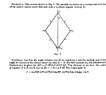# Trouble understanding coordinates for the Lagrangian

• I
p1ndol
Hello, I'm having some trouble understanding this solution provided in Landau's book on mechanics. I'd like to understand how they arrived at the infinitesimal displacement for the particles m1. I appreciate any kind of help regarding this problem, thank you!

#### Attachments

•Captura de Tela (65).png
16.8 KB · Views: 47
•Leo Liu

ergospherical
It's nothing more than Pythagoras applied to orthogonal infinitesimal displacements ##ad\theta## and ##a\sin{\theta} d\phi##, however if you want a (very slightly) more formal approach in terms of the holonomic basis...

if ##\phi## is held constant then ##\mathbf{E}_{\theta} = \dfrac{\partial \mathbf{r}}{\partial \theta} = a \hat{\boldsymbol{e}}_{\theta}## whilst if ##\theta## is held constant then ##\mathbf{E}_{\phi} = \dfrac{\partial \mathbf{r}}{\partial \phi} = a\sin{\theta} \hat{\boldsymbol{e}}_{\phi}##. Since ##\mathbf{E}_{\theta}## and ##\mathbf{E}_{\phi}## are orthogonal you have $$dl^2 = \displaystyle{\sum_i \sum_j }dx^i \mathbf{E}_i \cdot dx^j \mathbf{E}_j= {E_{\theta}}^2 d\theta^2 + {E_{\phi}}^2 d\phi^2 = a^2 d\theta^2 + a^2 \sin^2{\theta} d\phi^2$$

Last edited:
•vanhees71, Leo Liu and p1ndol
p1ndol
Thank you very much!

•ergospherical
Leo Liu
I think he did it in spherical coordinates. The infinitesimal motion of m1 can be disassembled into two parts; this disassembly is correct since the displacements in the two directions are small (meaning they are kinda linear) and orthogonal:

Displacement^2 caused by horizontal rotation ##\Omega##:
$$dl^2_{horizontal}=R^2(\Omega\mathrm{dt})^2=a^2\sin^2 \theta(\Omega\mathrm{dt})^2$$

Displacement^2 caused by the rotation of m1 about A in the plane of book:
$$v=r\omega\implies dl^2_{vertical}=(a\mathrm d{\theta})^2$$

Hope this helps.

•p1ndol
p1ndol
Thanks, you couldn't have been clearer!